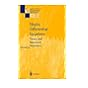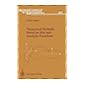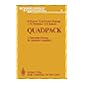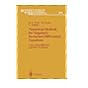Normal view

## Springer Series in Computational Mathematics Vol 24. Numerical methods for singularly perturbed differential equations : convection-diffusion and flow problems.

Additional authors: Stynes, Martin | Tobiska, LutzNo cover image available No cover image available No cover image available No cover image available 519.62 Springer Series in Computational Mathematics Vol 18. Elliptic differential equations : theory and numerical treatment. Translated from German by R. Fadiman and P.D.F. Ion. 519.62 Springer series in computational mathematics. Vol 20. Numerical methods based on Sinc and analytic functions. 519.62 Springer series in computational mathematics. Vol 1. Quadpack. A subroutine package for automatic integration. 519.62 Springer Series in Computational Mathematics Vol 24. Numerical methods for singularly perturbed differential equations : convection-diffusion and flow problems. 519.62 Finite element methods for Navier-Stokes equations : 519.62 Springer series in computational mathematics. Vol 17. LANCELOT. A Fortran package for large-scale nonlinear optimization ( Release A). 519.62 Springer series in computational mathematics. Vol 13. Numerical continuation methods : an introduction.